Question

# You are given the sample mean and the population standard deviation. Use this information to construct...

You are given the sample mean and the population standard deviation. Use this information to construct the​ 90% and​ 95% confidence intervals for the population mean. Interpret the results and compare the widths of the confidence intervals. If​ convenient, use technology to construct the confidence intervals.

A random sample of 45 home theater systems has a mean price of ​\$127.00. Assume the population standard deviation is ​\$19.20.

Construct a​ 90% confidence interval for the population mean.

The​ 90% confidence interval is :

The 95% confidence interval is: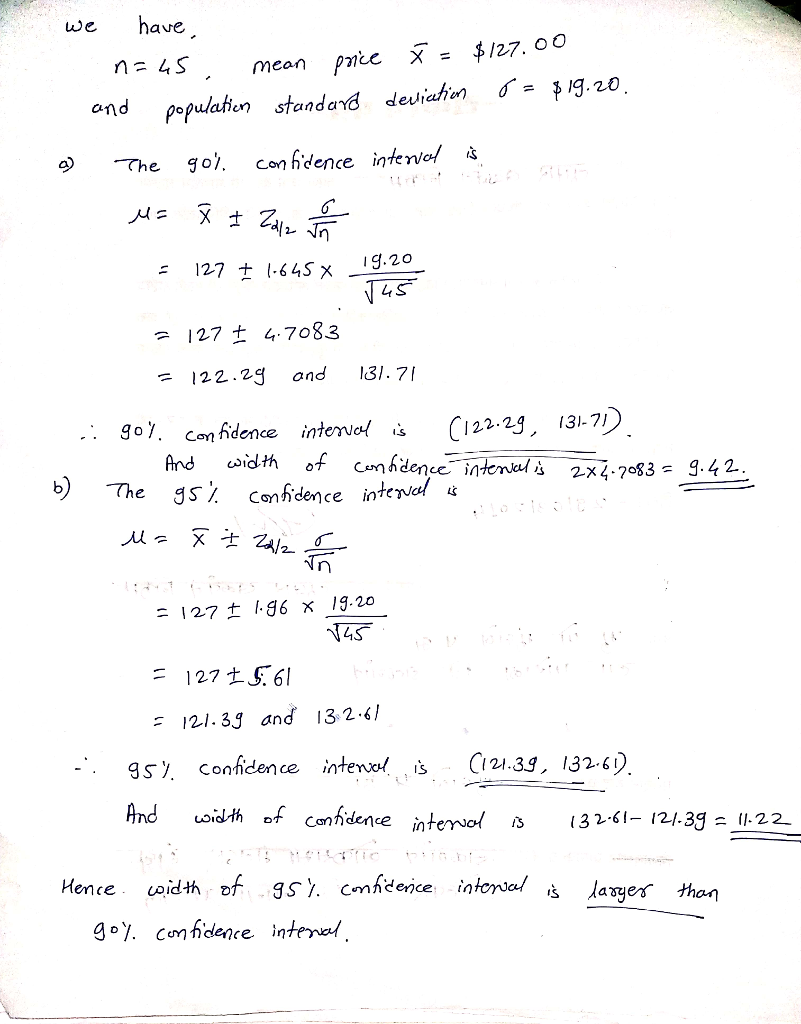#### Earn Coins

Coins can be redeemed for fabulous gifts.

Similar Homework Help Questions
• ### You are given the sample mean and the population standard deviation. Use this information to construct...

You are given the sample mean and the population standard deviation. Use this information to construct the​ 90% and​ 95% confidence intervals for the population mean. Interpret the results and compare the widths of the confidence intervals. If​ convenient, use technology to construct the confidence intervals. A random sample of 60 home theater systems has a mean price of ​\$113.00. Assume the population standard deviation is ​\$17.90. Construct a​ 90% confidence interval for the population mean

• ### You are given the sample mean and the population standard deviation. Use this information to construct...

You are given the sample mean and the population standard deviation. Use this information to construct the​ 90% and​ 95% confidence intervals for the population mean. Interpret the results and compare the widths of the confidence intervals. If​ convenient, use technology to construct the confidence intervals. A random sample of 60 home theater systems has a mean price of ​\$135.00 . Assume the population standard deviation is ​\$15.90 . A.) the 90% confidence interval is B.) the 95% confidence interval...

• ### You are given the sample mean and the population standard deviation. Use this information to construct...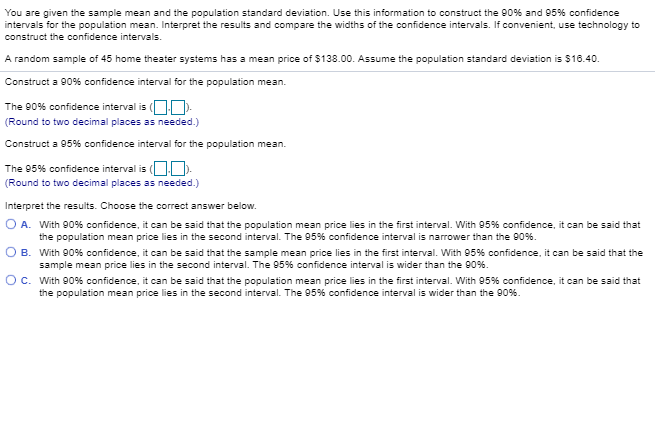You are given the sample mean and the population standard deviation. Use this information to construct the 90% and 95% confidence ntervals for the population mean. Interpret the results and compare the widths of the confidence intervals. If convenient, use technology to construct the confidence intervals A random sample of 45 home theater systems has a mean price of \$138.00. Assume the population standard deviation is 516.40. Construct a 90% confidence interval for the population mean. The 90% confidence interval...

• ### AM You are given the sample mean and the population standard deviation. Use this information to...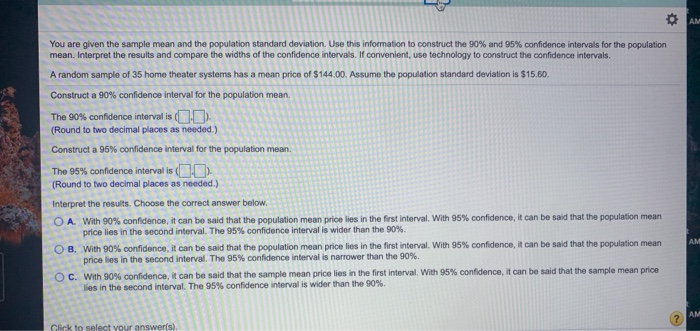AM You are given the sample mean and the population standard deviation. Use this information to construct the 90% and 95% confidence intervals for the population mean. Interpret the results and compare the widths of the confidence intervals. If convenient, use technology to construct the confidence intervals. A random sample of 35 home theater systems has a mean price of \$144.00. Assume the population standard deviation is \$15.60. Construct a 90% confidence interval for the population mean. The 90% confidence...

• ### You are given the sample mean and the population standard deviation. Use this information to construct...

You are given the sample mean and the population standard deviation. Use this information to construct the​ 90% and​ 95% confidence intervals for the population mean. Interpret the results and compare the widths of the confidence intervals. From a random sample of 49 business​ days, the mean closing price of a certain stock was ​\$113.41. Assume the population standard deviation is \$11.13. a) The​ 90% confidence interval is (?) - (?)

• ### You are given the sample mean and the population standard deviation. Use this information to construct...

You are given the sample mean and the population standard deviation. Use this information to construct the​ 90% and​ 95% confidence intervals for the population mean. Which interval is​ wider? If​ convenient, use technology to construct the confidence intervals. A random sample of 45 gas grills has a mean price of ​\$648.40 Assume the population standard deviation is ​\$56.50 The​ 90% confidence interval is

• ### You are given the sample mean and the population standard deviation. Use this information to construct...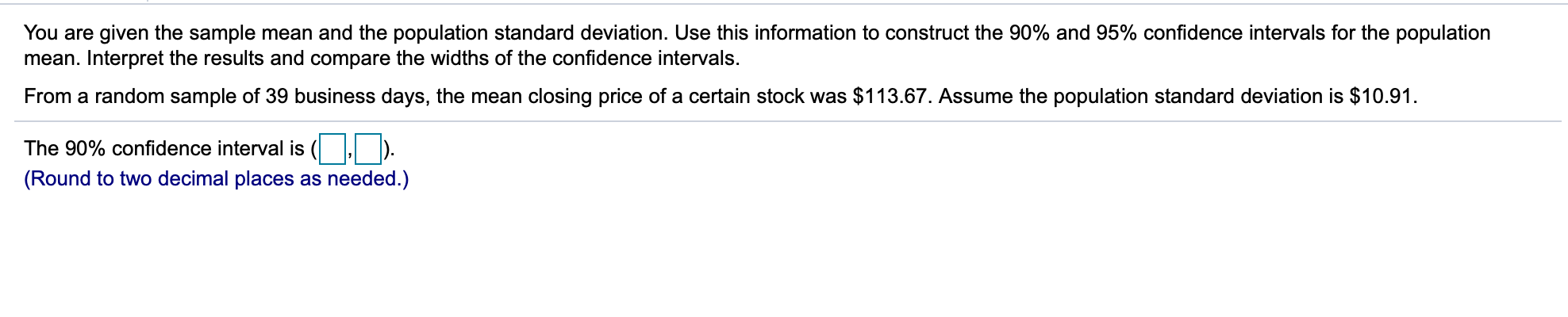You are given the sample mean and the population standard deviation. Use this information to construct the 90% and 95% confidence intervals for the population mean. Interpret the results and compare the widths of the confidence intervals. From a random sample of 39 business days, the mean closing price of a certain stock was \$113.67. Assume the population standard deviation is \$10.91. The 90% confidence interval is (1 , b). (Round to two decimal places as needed.)

• ### You are given the sample mean and the population standard deviation. Use this information to construct...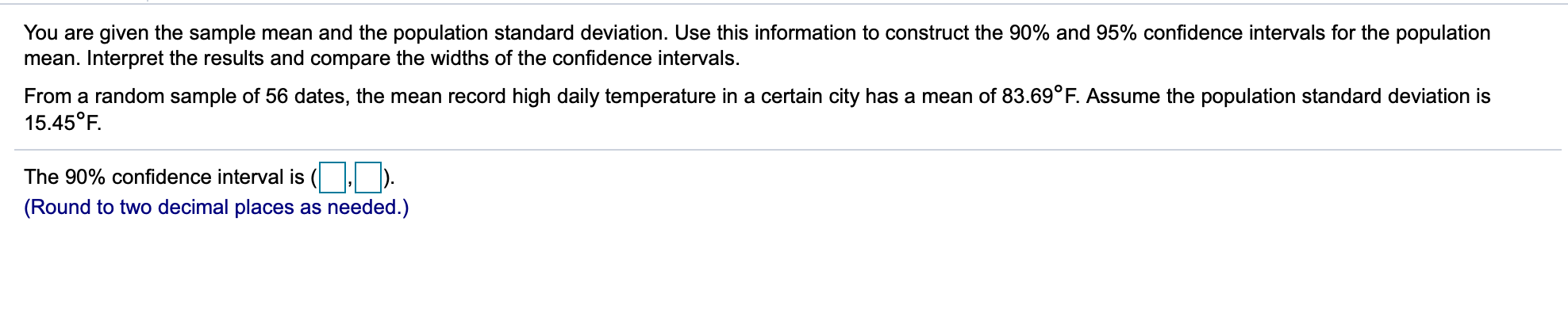You are given the sample mean and the population standard deviation. Use this information to construct the 90% and 95% confidence intervals for the population mean. Interpret the results and compare the widths of the confidence intervals From a random sample of 56 dates, the mean record high daily temperature in a certain city has a mean of 83.69°F. Assume the population standard deviation is 15.45°F. The 90% confidence interval is (Round to two decimal places as needed.)

• ### you are given the sample mean and the population and standard deviation. use this information to...

you are given the sample mean and the population and standard deviation. use this information to construct the 90% and 95% confidence intervals for the population mean. interpret the results and compare the widths of the confidence intervals. from a random sample of 77 dates, the mean record high daily temp in a certain city has a mean of 85.13 degrees F, assume the population standard deviation is 15.32 degrees F the 90% confidence interval is? the 95% confidence interval...

• ### You are given the sample mean and the population standard deviation. Use this information to construct...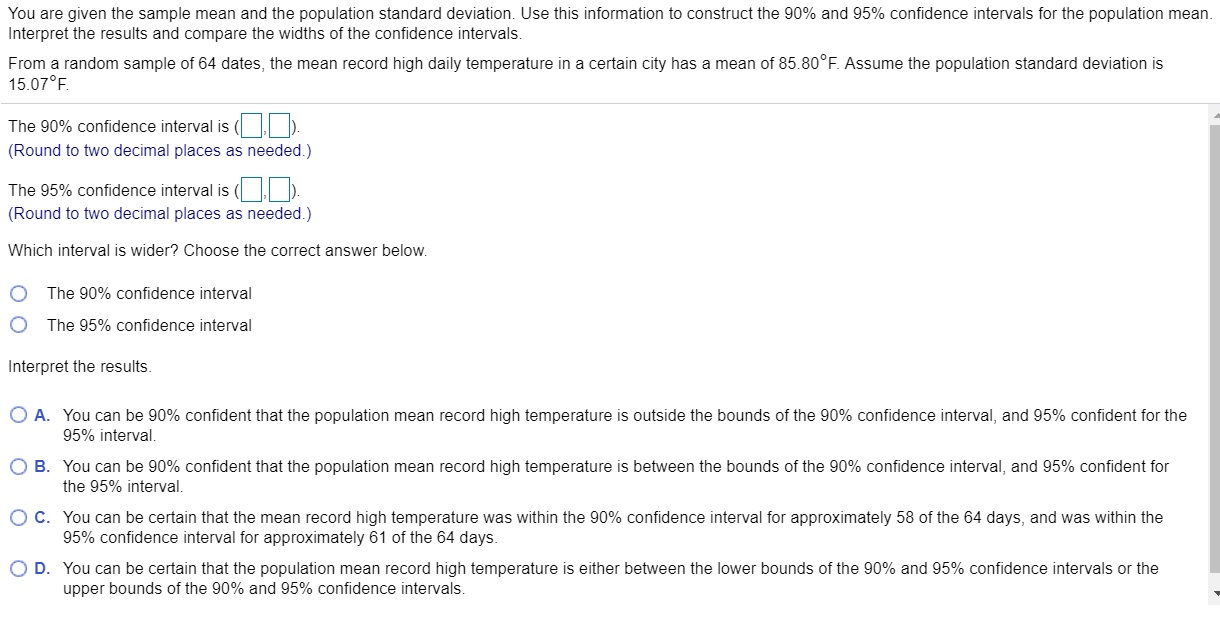You are given the sample mean and the population standard deviation. Use this information to construct the 90% and 95% confidence intervals for the population mean. Interpret the results and compare the widths of the confidence intervals. From a random sample of 64 dates, the mean record high daily temperature in a certain city has a mean of 85.80°F. Assume the population standard deviation is 15.07°F. The 90% confidence interval is (ID). (Round to two decimal places as needed.) The...